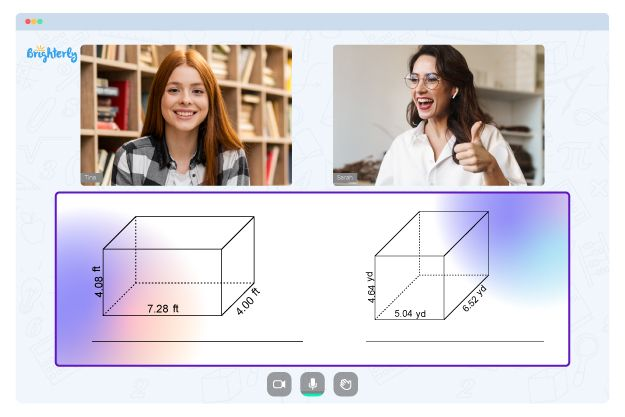# Triangle Sum Theorem Worksheets

Ever heard of the triangle sum theorem? Maybe you have. Or maybe you haven’t. However, it’s a lofty yet essential topic in mathematics. Learning this topic would involve memorizing a formula and applying it while solving exercises. Fortunately, our triangle sum theorem worksheet comes in pretty handy here. Let’s get into it, shall we?

## About the Triangle Angle Sum Worksheet

Before we delve any further, what is the triangle sum theorem? We know all about triangles; they’re pretty shapes with three sides. However, the triangle angle sum theorem states that the sum of the three interior angles in a triangle is always 180°. ALWAYS. It also goes further to state that the measure of the exterior angle is equal to the sum of its two opposite interior angles.

The formula for this theorem is pretty simple:

For a triangle ABC, we have A+B+C = 180°

The triangle sum theorem has varied applications and can even be extended to problems involving other polygons.

Math for Kids

Is Your Child Struggling With Math?
1:1 Online Math TutoringFortunately, the triangle angle sum theorem worksheet helps young learners to fully understand this concept. It’s an excellent resource for kids in Grades 5 through 8. It has a wide range of challenging resources that touch on both interior and exterior angles. Kids will learn how to apply the theorem formula in a variety of fun ways. The exercises are fun, challenging, and are in no way overwhelming for an average young learner.

This worksheet also comes with an extra perk: answers to all the exercises. The triangle angle sum worksheet answers helps kids cross-check their work and are quite handy for self-guided lessons. More importantly, they come with a logical explanation for each answer. This way, kids can easily learn and make corrections if they get a question wrong.### Triangle Sum Theorem Worksheets PDF

Triangle Sum Theorem Worksheet### Triangle Sum Theorem Worksheets PDF

Triangle Angle Sum Worksheet### Triangle Sum Theorem Worksheets PDF### Triangle Sum Theorem Worksheets PDF

Triangle Angle Sum Theorem Worksheet

The triangle sum theorem worksheet answers are a handy addition since they ease the learning process and offer an opportunity for independent learning. The worksheet itself also comes with a wide range of perks. For starters, kids gain a solid grasp of the theorem and its different applications.

The exercises are also filled with fun colorful illustrations, ensuring an interactive learning experience.

### More Worksheets

Struggling with Geometry?• Does your child struggle with understanding the concept of geometry?
• Try learning with an online tutor.

Is your child having difficulties mastering geometry concepts? An online tutor could provide the necessary help.